# About NBM v3.1 Text Bulletins (NBH,NBS,NBE,NBX)

The NBM text bulletins take data from the gridded NBM forecasts and generate data for weather stations from the closest usable grid point. (Note that these products are NOT calibrated to ground station observations, which is different from the MOS text bulletins.) Bulletins are available for over 3,000 stations in CONUS, Alaska, Hawaii and Puerto Rico for both land and marine stations, although not all elements may be available for all stations. All regions (including both land and marine stations) are included in the same product.

There are four NBM text bulletins, which cover different time scales and different elements. The products are as follows:

Product Name Product Type Time step Forecast Hours covered
NBH Hourly 1-Hourly Hours 1-25
NBS Short 3-Hourly Hours 6-66*
NBE Extended 12-Hourly Hours 24-192*
NBX Super-Extended 12-Hourly Hours 204-372* (data to 264*)  (continuation of NBE)

(*Actual forecast hours change according to cycle. Listed times are for 00Z and 12Z cycles.)

The bulletins consist of the following:

• The first line always consists of: the station call(short name), bulletin name, forecast date, and forecast cycle.
• The following 2-3 lines show the forecast valid date/time and/or model forecast hour. All forecast dates and times are listed in UTC time. (This differs from MOS bulletins, which are reported in Eastern local time.)
• The lines following the date/time consist of various weather elements. These elements vary by bulletin type, region, and sometimes cycle.
• Large values: any value which would be printed as >998 will be printed as 998
• ## Missing Data:

For all Elements, a value of 999 indicates missing data. If data are missing for all forecast hours, the line for that element will not be printed.

Example bulletins for each of the types (NBH,NBS,NBE,NBX) are listed below. At the bottom of the page, additional stations from different locations are displayed to show expected data at different regions (and at land vs. marine stations).

## NBS Example and Element Key

NBS Example Bulletin (CONUS, land station)Date/time lines

• DT (or blank) = forcast valid date (in UTC)
• UTC = forcast valid hour (in UTC)
• FHR = model forecast hour (number of hours forward from forecast date/cycle)

Elements

• X / N (or N/X) = 18-hour max / min (or min / max) temperature, degrees F. Min is between 00Z-18Z and recorded as 12Z; Max is between 12Z(current day)-06Z(next day) and recorded as 00Z(following day).
• TMP = temperature, degrees F
• DPT = dew point temperature, degrees F
• SKY = sky cover, percent
• WDR = wind direction, nearest tens degrees (northerly=36; easterly=9; calm=00)
• WSP = wind speed, knots (<0.5 knots will be listed as 0 (calm))
• GST = wind gust, knots
• P06 = 6-hour PoP, percent
• P12 = 12-hour PoP, percent
• Q06 = 6-hour QPF, 1/100 inches
• Q12 = 12-hour QPF, 1/100 inches
• T03 = 3-hour thunderstorm probability, percent
• T12 = 12-hour thunderstorm probability, percent
• PZR = conditional probability of freezing rain, percent
• PSN = conditional probability of snow, percent
• PPL = conditional probability of sleet / ice pellets, percent
• PRA = conditional probability of rain, percent
• S06 = 6-hour snow amount, 1/10 inches
• SLV = snow level, 100s feet MSL
• I06 = 6-hour ice amount, 1/100 inches
• CIG = ceiling height, 100s feet (>5000 ft reported to the nearest 1000 ft; 888=unlimited)
• VIS = visibility, miles
• LCB = lowest cloud base, 100s feet (>5000 ft reported to the nearest 1000 ft)
• SWH = significant wave height, feet (marine and near-water stations only)

(For additional information about NBM weather elements, see the Element Key)

## NBH Example and Element Key

NBH Example Bulletin (CONUS, land station)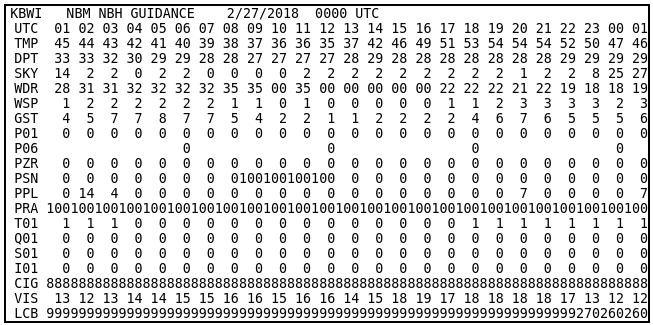Date/time lines

• UTC = forcast valid hour (in UTC) (date as in line 1, unless past 23Z - then the following date is valid)

Elements

• TMP = temperature, degrees F
• DPT = dew point temperature, degrees F
• SKY = sky cover, percent
• WDR = wind direction, nearest tens degrees (northerly=36; easterly=9; calm=00)
• WSP = wind speed, knots (<0.5 knots will be listed as 0 (calm))
• GST = wind gust, knots
• P01 = 1-hour PoP, percent
• P06 = 6-hour PoP, percent
• PZR = conditional probability of freezing rain, percent
• PSN = conditional probability of snow, percent
• PPL = conditional probability of sleet / ice pellets, percent
• PRA = conditional probability of rain, percent
• T01 = 1-hour thunderstorm probability, percent
• Q01 = 1-hour QPF, 1/100 inches
• S01 = 1-hour snow amount, 1/10 inches
• I01 = 1-hour ice amount, 1/100 inches
• CIG = ceiling height, 100s feet  (>5000 ft reported to the nearest 1000 ft; 888=unlimited)
• VIS = visibility, miles
• LCB = lowest cloud base, 100s feet (>5000 ft reported to the nearest 1000 ft)

(For additional information about NBM weather elements, see the Element Key)

## NBE Example and Element Key

NBE Example Bulletin (CONUS, land station)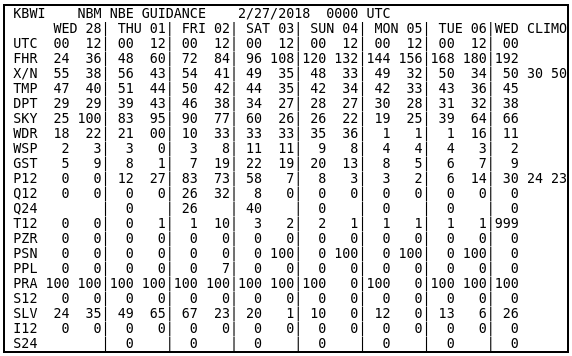Date/time lines

• (second line) = forcast valid dates (in UTC)
• UTC = forcast valid hour (in UTC)
• FHR = model forecast hour (number of hours forward from forecast date/cycle)

Elements

• X / N (or N/X) = 18-hour max / min (or min / max) temperature, degrees F. Min is between 00Z-18Z and recorded as 12Z; Max is between 12Z(current day)-06Z(next day) and recorded as 00Z(following day).
• TMP = temperature, degrees F
• DPT = dew point temperature, degrees F
• SKY = sky cover, percent
• WDR = wind direction, nearest tens degrees (northerly=36; easterly=9; calm=00)
• WSP = wind speed, knots (<0.5 knots will be listed as 0 (calm))
• GST = wind gust, knots
• P12 = 12-hour PoP, percent
• Q12 = 12-hour QPF, 1/100 inches
• Q24 = 24-hour QPF, 1/100 inches
• T12 = 12-hour thunderstorm probability, percent
• PZR = conditional probability of freezing rain, percent
• PSN = conditional probability of snow, percent
• PPL = conditional probability of sleet / ice pellets, percent
• PRA = conditional probability of rain, percent
• S12 = 12-hour snow amount, 1/10 inches
• SLV = snow level, 100s feet MSL
• I12 = 12-hour ice amount, 1/100 inches
• S24 = 24-hour snow amount, 1/10 inches
• SWH = significant wave height, feet (marine and near-water stations only)

(For additional information about NBM weather elements, see the Element Key)

CLIMO

For 00Z and 12Z cycles, Max/Min Temperature and 12-hour PoP, a reference climatology is displayed. These numbers are the climatological normal max and min temperature for the given date, based on the 1961-1990 NCDC data. For PoP12, the CLIMO displays the normal observed relative frequencies of 0.01 inches or more of precipitation during the 96-108 and 108-120h (00z) or 84-96 and 96-108 h (12Z) periods. The technique generating these climatologies is the same as for MEX bulletins, and detailed information can be found in MDL TPB 05-07. These numbers are the same for both NBE and NBX of a given date.

## NBX Example and Element Key

NBX Example Bulletin (CONUS, land station)Date/time lines

• (second line) = forcast valid dates (in UTC)
• UTC = forcast valid hour (in UTC)
• FHR = model forecast hour (number of hours forward from forecast date/cycle)

Elements

• X / N (or N/X) = 18-hour max / min (or min / max) temperature, degrees F. Min is between 00Z-18Z and recorded as 12Z; Max is between 12Z(current day)-06Z(next day) and recorded as 00Z(following day).
• TMP = temperature, degrees F
• DPT = dew point temperature, degrees F
• SKY = sky cover, percent
• WDR = wind direction, nearest tens degrees (northerly=36; easterly=9; calm=00)
• WSP = wind speed, knots (<0.5 knots will be listed as 0 (calm))
• GST = wind gust, knots
• P12 = 12-hour PoP, percent
• Q12 = 12-hour QPF, 1/100 inches
• Q24 = 24-hour QPF, 1/100 inches
• T12 = 12-hour thunderstorm probability, percent
• PZR = conditional probability of freezing rain, percent
• PSN = conditional probability of snow, percent
• PPL = conditional probability of sleet / ice pellets, percent
• PRA = conditional probability of rain, percent
• S12 = 12-hour snow amount, 1/10 inches
• SLV = snow level, 100s feet MSL
• I12 = 12-hour ice amount, 1/100 inches
• S24 = 24-hour snow amount, 1/10 inches
• SWH = significant wave height, feet (marine and near-water stations only)

(For additional information about NBM weather elements, see the Element Key)

CLIMO

For 00Z and 12Z cycles, Max/Min Temperature and 12-hour PoP, a reference climatology is displayed. These numbers are the climatological normal max and min temperature for the given date, based on the 1971-2000 NCDC data. For PoP12, the CLIMO displays the normal observed relative frequencies of 0.01 inches or more of precipitation during the 96-108 and 108-120h (00z) or 84-96 and 96-108 h (12Z) periods. The technique generating these climatologies is the same as for MEX bulletins, and detailed information can be found in MDL TPB 05-07. These numbers are the same for both NBE and NBX of a given date.

## Additional Examples

### NBS

NBS Example Bulletin, CONUS (Marine)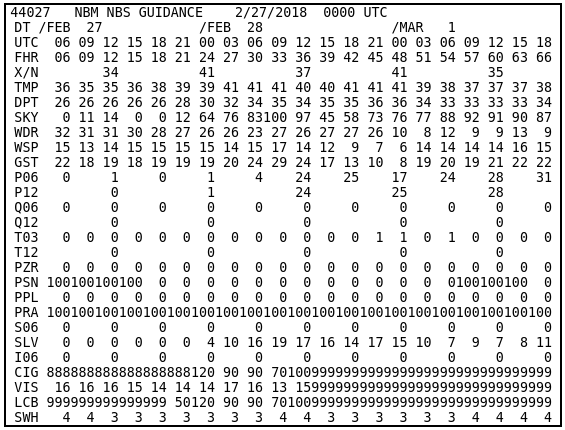NBS Example Bulletin, Alaska (Land)NBS Example Bulletin, Alaska (Marine)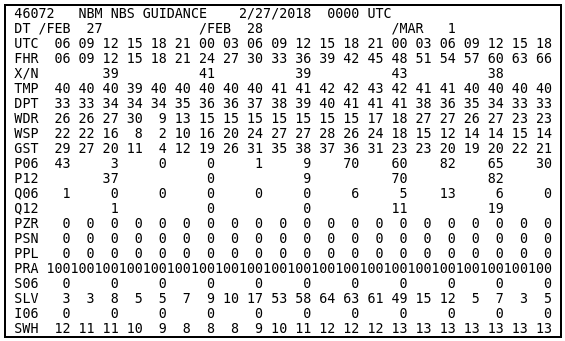NBS Example Bulletin, Hawaii (Land)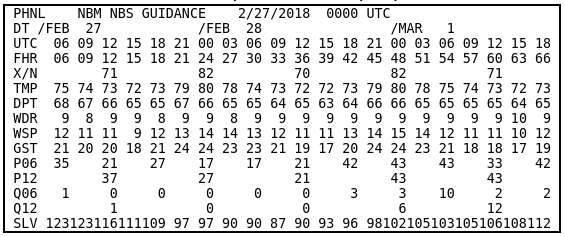NBS Example Bulletin, Hawaii (Marine)NBS Example Bulletin, Puerto Rico (Land)NBS Example Bulletin, Puerto Rico (Marine)